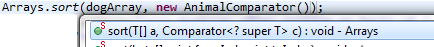### Program to understand Comparator of superclass can be used by subclasses

Animal is superclass of Dog and Lion.
dogArray is array of dogs.
AnimalComparator is a Comparator of Animal class [ class AnimalComparator implements Comparator<Animal> ]

We will be using Arrays.sort(dogArray, new AnimalComparator()); in program.

Method definition of sort method in java.util.Arrays is -
 public static void sort(T[] a, Comparator c)

So, what do you mean by second parameter  Comparator<? super T> cLet’s understand first parameter -
 T[] a dogArray has been passed as parameter which is array of Dog, and Dog extends Animal. So, T is Dog, and T[] is dogArray.

Now, let’s understand second parameter -
 Comparator c In the program, following 2 lines have been used > class Dog extends Animal (Animal is superclass of Dog), class AnimalComparator implements Comparator (AnimalComparator is a Comparator of Animal class but it can be used by subclasses (i.e. Dog and Lion) as well.)

Full program -
 import java.util.Arrays; import java.util.Comparator; /** * Animal class */ class Animal{    Integer height; } /** * Lion class */ class Lion extends Animal{    Lion(Integer height){           this.height=height;    }       @Override    public String toString() {           return "Lion [height=" + height + "]";    } } /** * Dog class */ class Dog extends Animal{    Dog(Integer height){           this.height=height;    }      @Override    public String toString() {           return "Dog [height=" + height + "]";    } } /** * Comparator to sort animal's height in ascending order. */ class AnimalComparator implements Comparator {    @Override    public int compare(Animal o1, Animal o2) {           //using Comparator to sort animal's height in ascending order.           return o1.height.compareTo(o2.height);    } } /** * @author AnkitMittal * Copyright (c), AnkitMittal JavaMadeSoEasy.com * Main class */ public class ArraysSort {    public static void main(String...a){ System.out.println("-----Sorting Lion's height -- using AnimalComparator-----");       Lion lion1=new Lion(3);       Lion lion2=new Lion(1);       Lion lion3=new Lion(2);       Lion lionArray[]={lion1, lion2, lion3};                  System.out.print(" lionArray before sorting : \n");           for (Animal animal : lionArray) {                  System.out.print(animal + "  ");           }           Arrays.sort(lionArray, new AnimalComparator());           System.out.println("\n\n lionArray after sorting on basis of "                        + "height(ascending order) : ");           for (Animal animal : lionArray) {                  System.out.print(animal + "  ");           } System.out.println("\n\n\n------Sorting Dog's height -- using AnimalComparator----");              Dog dog1=new Dog(2);       Dog dog2=new Dog(1);       Dog dog3=new Dog(3);       Dog dogArray[]={dog1, dog2, dog3};                  System.out.print(" dogArray before sorting : \n");           for (Animal animal : dogArray) {                  System.out.print(animal + "  ");           }           Arrays.sort(dogArray, new AnimalComparator());           System.out.println("\n\n dogArray after sorting on basis of "                        + "height(ascending order) : ");           for (Animal animal : dogArray) {                  System.out.print(animal + "  ");           }           } } /*OUTPUT ----------Sorting Lion's height -- using AnimalComparator---------- lionArray before sorting : Lion [height=3]  Lion [height=1]  Lion [height=2] lionArray after sorting on basis of height(ascending order) : Lion [height=1]  Lion [height=2]  Lion [height=3] --------Sorting Dog's height -- using AnimalComparator--------- dogArray before sorting : Dog [height=2]  Dog [height=1]  Dog [height=3] dogArray after sorting on basis of height(ascending order) : Dog [height=1]  Dog [height=2]  Dog [height=3] */